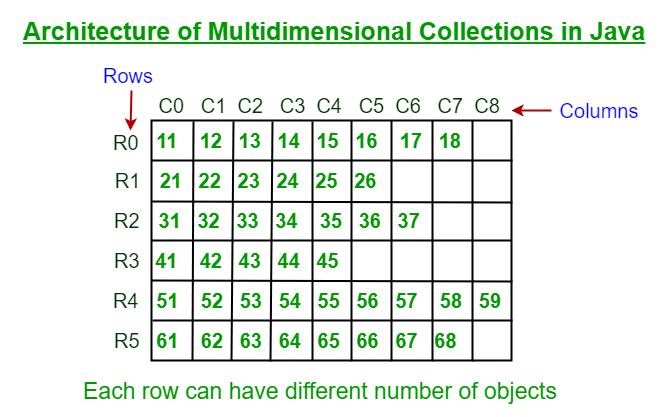Open in App
Not now

# Multidimensional Collections in Java

• Difficulty Level : Easy
• Last Updated : 13 Sep, 2022

In Java, we have a Collection framework that provides functionality to store a group of objects. This is called a single-dimensional ArrayList where we can have only one element in a row. Geek but what if we want to make a multidimensional ArrayList, for this functionality for which we do have Multidimensional Collections (or Nested Collections) in Java.

Multidimensional Collections (or Nested Collections) is a collection of groups of objects where each group can have any number of objects dynamically. Hence, here we can store any number of elements in a group whenever we want.Illustration:

```Single dimensional ArrayList :
[121, 432, 12, 56, 456, 3, 1023]
[Apple, Orange, Pear, Mango]```

Syntax:

`ArrayList <Object> x = new ArrayList <Object>();`

## Need for Multidimensional Collections

Unlike Arrays, we are not bound with the size of any row in Multidimensional collections. Therefore, if we want to use a Multidimensional architecture where we can create any number of objects dynamically in a row, then we should go for Multidimensional collections in java.

Syntax: Multidimensional Collections

`ArrayList<ArrayList<Object>> a = new ArrayList<ArrayList<Object>>();`

Illustration:

`Multidimensional ArrayList: [[3, 4], [12, 13, 14, 15], [22, 23, 24], ]`

Let us quickly peek onto add() method for multidimensional ArrayList which are as follows:

• boolean add( ArrayList<Object> e): It is used to insert elements in the specified collection.
• void add( int index, ArrayList<Object> e): It is used to insert the elements at the specified position in a Collection.

Example 1:

## Java

 `// Java Program to Illustrate Multidimensional ArrayList` `// Importing required classes``import` `java.util.*;` `// Main class``// MultidimensionalArrayList``class` `GFG {` `    ``// Method 1``    ``// To create and return 2D ArrayList``    ``static` `List create2DArrayList()``    ``{` `        ``// Creating a 2D ArrayList of Integer type``        ``ArrayList > x``            ``= ``new` `ArrayList >();` `        ``// One space allocated for R0``        ``x.add(``new` `ArrayList());` `        ``// Adding 3 to R0 created above x(R0, C0)``        ``x.get(``0``).add(``0``, ``3``);` `        ``// Creating R1 and adding values``        ``// Note: Another way for adding values in 2D``        ``// collections``        ``x.add(``            ``new` `ArrayList(Arrays.asList(``3``, ``4``, ``6``)));` `        ``// Adding 366 to x(R1, C0)``        ``x.get(``1``).add(``0``, ``366``);` `        ``// Adding 576 to x(R1, C4)``        ``x.get(``1``).add(``4``, ``576``);` `        ``// Now, adding values to R2``        ``x.add(``2``, ``new` `ArrayList<>(Arrays.asList(``3``, ``84``)));` `        ``// Adding values to R3``        ``x.add(``new` `ArrayList(``            ``Arrays.asList(``83``, ``6684``, ``776``)));` `        ``// Adding values to R4``        ``x.add(``new` `ArrayList<>(Arrays.asList(``8``)));` `        ``// Appending values to R4``        ``x.get(``4``).addAll(Arrays.asList(``9``, ``10``, ``11``));` `        ``// Appending values to R1, but start appending from``        ``// C3``        ``x.get(``1``).addAll(``3``, Arrays.asList(``22``, ``1000``));` `        ``// This method will return 2D array``        ``return` `x;``    ``}` `    ``// Method 2``    ``// Main driver method``    ``public` `static` `void` `main(String args[])``    ``{``        ``// Display message prior for better readability``        ``System.out.println(``"2D ArrayList :"``);` `        ``// Printing 2D ArrayList by calling Method 1``        ``System.out.println(create2DArrayList());``    ``}``}`

Output

```2D ArrayList :
[, [366, 3, 4, 22, 1000, 6, 576], [3, 84], [83, 6684, 776], [8, 9, 10, 11]]```

Now let us see the same implementation of multidimensional LinkedHashSet and in order to show how it behaves differently. Similarly, we can implement any other Collection as Multidimensional Collection as depicted below:

Syntax:

`HashSet< HashSet<Object> > a = new HashSet< HashSet<Object> >(); `

Note: LinkedHashSet class contains unique elements & maintains insertion order. Therefore, in Multidimensional LinkedHashSet uniqueness will be maintained inside rows also.

Example 2:

## Java

 `// Java Program to Illustrate Multidimensional LinkedHashSet` `// Importing required classes``import` `java.util.*;` `// Main class``// Multidimensional LinkedHashSet``class` `GFG {` `    ``// Method 1``    ``// To create and return 2D LinkedHashSet``    ``static` `Set create2DLinkedHashSet()``    ``{``        ``// Creating an empty 2D LinkedHashSet``        ``LinkedHashSet > x``            ``= ``new` `LinkedHashSet >();` `        ``// Creating R0``        ``x.add(``new` `LinkedHashSet(``            ``Arrays.asList(``"Apple"``, ``"Orange"``)));` `        ``// Creating R1, here "Coffee" will be considered as``        ``// only one object to maintain uniqueness``        ``x.add(``new` `LinkedHashSet(Arrays.asList(``            ``"Tea"``, ``"Coffee"``, ``"Milk"``, ``"Coffee"``, ``"Water"``)));` `        ``// Creating R2``        ``x.add(``new` `LinkedHashSet(``            ``Arrays.asList(``"Tomato"``, ``"Potato"``, ``"Onion"``)));` `        ``// Creating R3 but it will not be added as it``        ``// contains the same items as R2` `        ``// Note: LinkedHashSet inserts only unique items` `        ``x.add(``new` `LinkedHashSet(``            ``Arrays.asList(``"Tomato"``, ``"Potato"``, ``"Onion"``)));` `        ``// Returning multidimensional LinkedHashSet``        ``return` `x;``    ``}` `    ``// Method 2``    ``// Main driver method``    ``public` `static` `void` `main(String[] args)``    ``{``        ``// Display message for better readability``        ``System.out.println(``"2D LinkedHashSet :"``);` `        ``// Printing 2D LinkedHashSet by``        ``// calling method 1``        ``System.out.println(create2DLinkedHashSet());``    ``}``}`

Output

```2D LinkedHashSet :
[[Apple, Orange], [Tea, Coffee, Milk, Water], [Tomato, Potato, Onion]]```

My Personal Notes arrow_drop_up